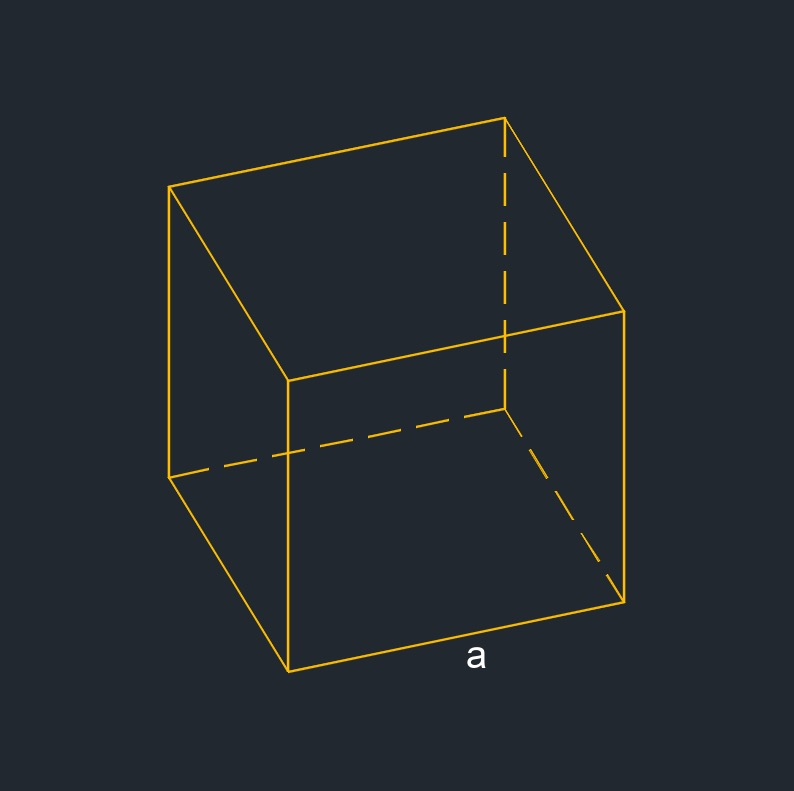# Cube

on . Posted in Solid Geometry

•Cube (a three-dimensional figure) is a regular polyhedron with square faces.
• All edges are the same length.
• All faces are squares
• Diagonal is a line from one vertices to another that is non adjacent.
• Circumscribed sphere is a polyhedron is a sphere that contains the polyhedron and touches each of the ployhedron's vertices.
• Inscribed sphere - A convex polyhedron is a sphere that is contained within the polyhedron and tangent to each of the polyhedron's faces.
• Midsphere is a polyhedron is a sphere that is tangent to every edge of the polyhedron.
• 4 base diagonals
• 24 face diagonals
• 4 space diagonals
• 12 edges
• 6 faces
• 8 vertex## Cube Circumscribed Sphere Radius formula

$$\large{ R = a \; \frac{ \sqrt {3} }{2} }$$
Symbol English Metric
$$\large{ R }$$ = circumscribed sphere radius $$\large{ in }$$  $$\large{ mm }$$
$$\large{ a }$$ = edge $$\large{ in }$$ $$\large{ mm }$$## Circumscribed Sphere Volume of a Cube formula

$$\large{ C_v = \frac{3}{4} \; \pi \; \left( a\; \frac{ \sqrt {3} }{2} \right) ^3 }$$
Symbol English Metric
$$\large{ C_v }$$ = circumscribed sphere volume $$\large{ in^3 }$$  $$\large{ mm^3 }$$
$$\large{ a }$$ = edge $$\large{ in }$$ $$\large{ mm }$$
$$\large{ \pi }$$ = Pi $$\large{3.141 592 653 ...}$$

## Edge of a Cube formulas

$$\large{ a = \sqrt { \frac { A_s } { 6 } } }$$

$$\large{ a = V^{1/3} }$$

$$\large{ a = \sqrt { 3 } \; \frac { D' } {3} }$$

Symbol English Metric
$$\large{ a }$$ = edge $$\large{ in }$$ $$\large{ mm }$$
$$\large{ D' }$$ = space diagonal $$\large{ in }$$ $$\large{ mm }$$
$$\large{ A_s }$$ = surface face area $$\large{ in }$$ $$\large{ mm }$$
$$\large{ V }$$ = volume $$\large{ in^3 }$$ $$\large{ mm^3 }$$## Face Area of a Cube formula

$$\large{ A_{area} = a^2 }$$
Symbol English Metric
$$\large{ A_{area} }$$ = face area $$\large{ in^2 }$$  $$\large{ mm^2 }$$
$$\large{ a }$$ = edge $$\large{ in }$$ $$\large{ mm }$$

## Inscribed Radius of a Cube formula

$$\large{ r = \frac{a}{2} }$$
Symbol English Metric
$$\large{ r }$$ = inside radius $$\large{ in }$$  $$\large{ mm }$$
$$\large{ a }$$ = edge $$\large{ in }$$ $$\large{ mm }$$

## Inscribed Sphere Volume of a Cube formula

$$\large{ I_v = \frac{3}{4} \; \pi \; \left( \frac{ a }{2} \right) ^3 }$$
Symbol English Metric
$$\large{ I_v }$$ = inscribed sphere volume $$\large{ in^3 }$$  $$\large{ mm^3 }$$
$$\large{ a }$$ = edge $$\large{ in }$$ $$\large{ mm }$$
$$\large{ \pi }$$ = Pi $$\large{3.141 592 653 ...}$$

## Midsphere Radius of a Cube formula

$$\large{ r_m = \frac{a}{2} \sqrt {2} }$$
Symbol English Metric
$$\large{ r_m }$$ = midsphere radius $$\large{ in }$$  $$\large{ mm }$$
$$\large{ a }$$ = edge $$\large{ in }$$ $$\large{ mm }$$

## Space Diagonal of a Cube formula

$$\large{ D' = \sqrt {3} \;a }$$
Symbol English Metric
$$\large{ D' }$$ = space diagonal $$\large{ in }$$  $$\large{ mm }$$
$$\large{ a }$$ = edge $$\large{ in }$$ $$\large{ mm }$$

## Surface face Area of a Cube formula

$$\large{ A_s = 6\;a^2 }$$
Symbol English Metric
$$\large{ A_s }$$ = surface face area $$\large{ in^2 }$$  $$\large{ mm^2 }$$
$$\large{ a }$$ = edge $$\large{ in }$$ $$\large{ mm }$$## Surface to volume ratio of a Cube formula

$$\large{ S_v = \frac{6}{a} }$$
Symbol English Metric
$$\large{ S_v }$$ = surface to volume ratio $$\large{ in^3 }$$  $$\large{ mm^3 }$$
$$\large{ a }$$ = edge $$\large{ in }$$ $$\large{ mm }$$## Volume of a Cube formula

$$\large{ V = a^3 }$$
Symbol English Metric
$$\large{ V }$$ = volume $$\large{ in^3 }$$  $$\large{ mm^3 }$$
$$\large{ a }$$ = edge $$\large{ in }$$ $$\large{ mm }$$## Weight of a Cube formula

$$\large{ m = a^3 \rho }$$
Symbol English Metric
$$\large{ m }$$ = mass $$\large{ lbm }$$  $$\large{ kg }$$
$$\large{ \rho }$$   (Greek symbol rho) = density $$\large{\frac{lbm}{ft^3}}$$ $$\large{\frac{kg}{m^3}}$$
$$\large{ a }$$ = edge $$\large{ in }$$ $$\large{ mm }$$Tags: Volume